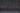# What is a Gaussian process?

Date September 22, 2020
Author James Leedham

A Gaussian process (GP) is a probabilistic AI technique that can generate accurate predictions from low volumes of historical data and other sources of information, irrespective of noise in the signal.

It builds upon the principled mathematical properties of the Gaussian (or 'normal') distribution, whose bell curve elegantly describes the harmony and symmetry inherent in numerous natural and social phenomena.

In general, a machine learning model is a mathematical representation of a real-world process or activity. A model enables you to analyse the mechanics of the system under observation in order to understand it better, as well as to predict how it might behave in the future. This enables you to make more informed decisions, or even to automate the decision-making process.

Because it is probabilistic, a GP model doesn’t generate just a single prediction but rather describes all the possible outcomes, and calculates a likelihood for each of them. This means you could, for example, determine that there is a 90% chance a particular answer will turn out to be correct, and so place an appropriate level of trust in that prediction, and make a more informed decision.

A GP works by examining a ‘prior’, which encapsulates expert knowledge of meaning or trends in existing data, and using Bayesian inference to compute a ‘posterior’, which is a probabilistic description of all the possible outcomes that are compatible with both the prior and the data. Each prediction is assigned a confidence interval, which constitutes the bounds of how mathematically likely the model believes it to be.

A GP is particularly data-efficient because the application of expert knowledge provides a great deal of information to the model. As a result, models can be trained on small quantities of readily available data, and can generate predictions in real time on live data, without prohibitive time, energy and hardware costs.

The ability to calculate confidence intervals constitutes the real power and utility of a GP. A set of possible outcomes might itself be a Gaussian distribution, with a mean (average) that constitutes the model’s prediction, and a variance that describes how far the outcomes deviate from that mean. If the variance is narrow, uncertainty is correspondingly low, and the model can be confident in its prediction. If the variance is wide, uncertainty is correspondingly high, and the model must transparently acknowledge its lack of confidence.

Share
,,